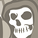# QlikView App Dev

Discussion Board for collaboration related to QlikView App Development.

Announcements
Now Live: Qlik Sense SaaS Simplified Authoring – Analytics Creation for Everyone: READ DETAILS
cancel
Showing results for
Did you mean:Contributor II

## Identify Top 3 in Territory Based on selection with Set Analysis

New to Qlikview and need some support with the Set Analysis.

There are 4 regions and each region has some dealers. If user select Dealer A and Dealer A belongs to Region North. Then identify the Top 3 dealers in Region North. And make some calculation with Top 3 dealers and compare to Dealer A. Top 3 should be based on the rank of (Revenue/Qty). Qty = Count(PRODUCTID).

Tried 2 solutions but they did not work:

Solution 1:

Create a variable Top_3_Dealers.

Make a aggregation of Revenue/Qty for each dealer. And rank based on the result. if rank <= 3, concatenate the dealer ID together. Expected result: Top_3_Dealers = 'B', 'C', 'D'

=mid(concat({<REGION={\$(=MaxString(REGION))}>}if(aggr({<REGION={\$(=MaxString(REGION))}>}rank(sum({<REGION={\$(=MaxString(REGION))}>}TOTAL_REVENUE)/count({<REGION={\$(=MaxString(REGION))}>}PRODUCTID)), DEALERID)<=3, chr(39) & DEALERID & chr(39) & ',')),1, Len(concat({<REGION={\$(=MaxString(REGION))}>}if(aggr({<REGION={\$(=MaxString(REGION))}>}rank(sum({<REGION={\$(=MaxString(REGION))}>}TOTAL_REVENUE)/count({<REGION={\$(=MaxString(REGION))}>}PRODUCTID)), DEALERID)<=3, chr(39) & DEALERID & chr(39) & ','))) - 1 )

And then calculate the weighted average of the Top 3 dealers:

=sum({<DEALERID={\$(=Top_3_Dealers)}>}TOTAL_REVENUE)

When only REGION is selected and DEALERID is not selected, it would work as expected. However if DEALERID is selected, the Top_3_Dealers always display the selected dealer.

Solution 2:

Create a variable CurrentRegion, the definition is:

=MaxString(REGION)

Create a variable Top_3_Dealers similar to solution 1 but replace the MaxString(REGION) with

=mid(concat({<REGION={\$(=CurrentRegion))}>}if(aggr({<REGION={\$(=CurrentRegion)}>}rank(sum({<REGION={\$(=CurrentRegion)}>}TOTAL_REVENUE)/count({<REGION={\$(=CurrentRegion)}>}PRODUCTID)), DEALERID)<=3, chr(39) & DEALERID & chr(39) & ',')),1, Len(concat({<REGION={\$(=MaxString(REGION))}>}if(aggr({<REGION={\$(=CurrentRegion)}>}rank(sum({<REGION={\$(=CurrentRegion)}>}TOTAL_REVENUE)/count({<REGION={\$(=CurrentRegion)}>}PRODUCTID)), DEALERID)<=3, chr(39) & DEALERID & chr(39) & ','))) - 1 )

Same problem.

Could anybody help? Cannot upload the qvw file because of company regulations. Probably just use every simple aggregation to explain the idea(e.g. use sum(sales) as the ranking basis).

The set analysis worked when I was doing the previous month calculation:

=aggr(sum({<RETAIL_MONTH={\$(=max(RETAIL_MONTH)-1)}>}TOTAL_REVENUE)/count({<RETAIL_MONTH={\$(=max(RETAIL_MONTH)-1)}>}PRODUCTID), DEALERID)

But why it's not working here?

Jason

1 Solution

Accepted SolutionsContributor II
Author

Thanks a lot!

In actual situation, we have a different field similar to PRODUCTID, like the each item has an identification number. And the field is not easy to understand for others so I changed it to PRODUCTID, however it seems more confusingI found out a workable solution:

=concat({<DEALERID=, REGION=p(REGION)>}if(aggr(rank(sum({<DEALERID=, REGION=p(REGION)>}TOTAL_REVENUE)/count({<DEALERID=, REGION=p(REGION)>}PRODUCTID)), DEALERID)<=3, chr(39) & DEALERID& chr(39) & ','))

2 RepliesMVP

Try something like this:

concat({<DEARERID={"=Rank(Sum({<REGION=P(REGION)>}TOTAL_REVENUE)/COUNT({<REGION=P(REGION)>}) PRODUCTID )<=3"}, REGION=P(REGION)>} DEALERID, ',' )

Are you sure the quantity is Count(PRODUCTID)? It will count the number of records that contain a PRODUCTID. Does that really equal the quantity?

talk is cheap, supply exceeds demandContributor II
Author

Thanks a lot!

In actual situation, we have a different field similar to PRODUCTID, like the each item has an identification number. And the field is not easy to understand for others so I changed it to PRODUCTID, however it seems more confusingI found out a workable solution:

=concat({<DEALERID=, REGION=p(REGION)>}if(aggr(rank(sum({<DEALERID=, REGION=p(REGION)>}TOTAL_REVENUE)/count({<DEALERID=, REGION=p(REGION)>}PRODUCTID)), DEALERID)<=3, chr(39) & DEALERID& chr(39) & ','))